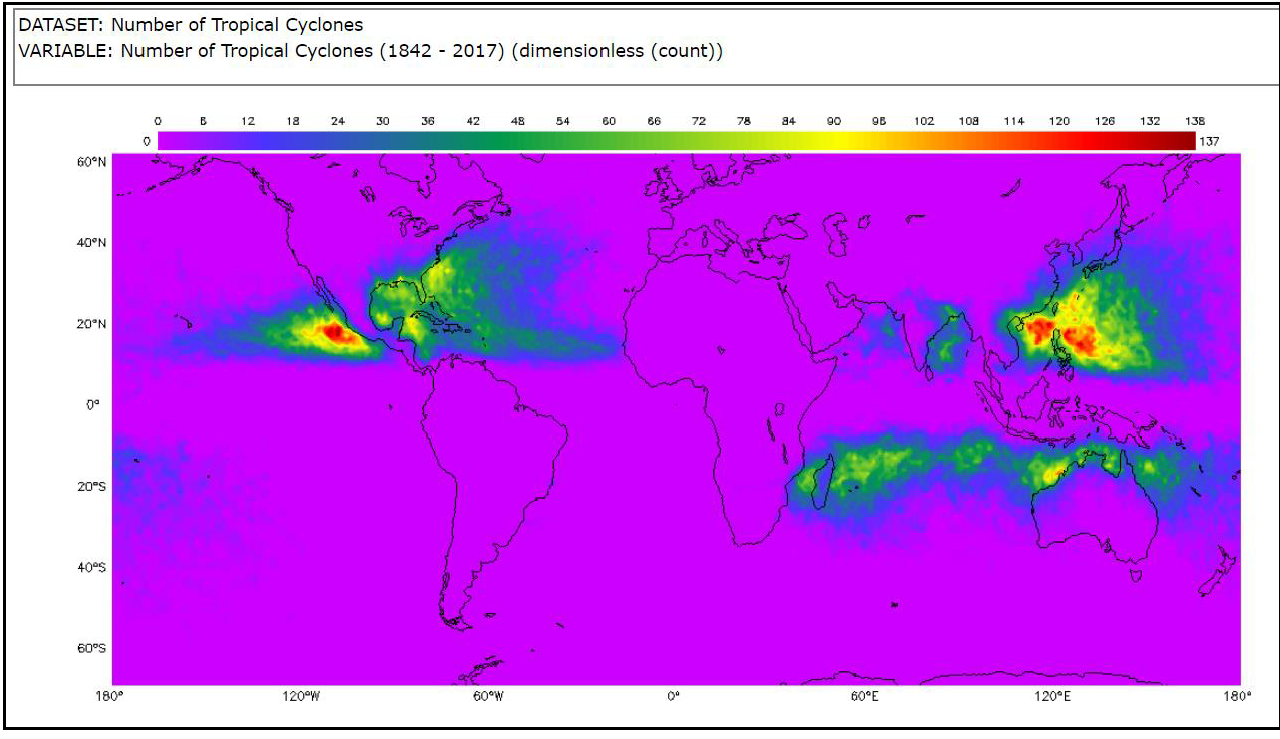## Tropical Cyclone Counts Scatter Plot

Students will analyze and interpret a scatter plot and evaluate the spread of the data. Students will compare it with a different visualization of the data to see how the two compare. Students will discuss the limitations of the two types of data displays and develop questions to help them develop data literacy skills. Students can create the scatter plot or use the one provided. This is part of the Tropical Cyclone Counts Graphing Bundle and can be completed independently or with the other activities in the bundle.

### Mini Lesson

#### Introduce and Post the Driving Question

Which data display is most useful for determining the risk of a tropical cyclone in a given area and preparing an effective emergency plan?

#### Analyze Tropical Cyclone Map Image

1. If students already completed the Tropical Cyclone Counts Histogram or Tropical Cyclone Count Box Plot mini lesson, quickly review the patterns they identified, use the maps that were previously marked and continue to the Review Scatter Plots section.

2. Tropical cyclones are sometimes called hurricanes or typhoons. The image is a model of the number of tropical cyclones around the world from 1842 – 2017. It was generated in the My NASA Data Earth System Data Explorer1. #### Think-Pair-Share Questions

1. Examine the map at 120° West. What do you see at the different latitudes?

2. Circle the area on the map at 120° West with the most tropical cyclones.

3. Put a box around the areas on the map at 120° West between the equator and 40 degrees North with the lowest number of tropical cyclones.

4. Describe the patterns you see.

5. Have students share what they discussed.

#### Review Scatter Plots

Option 1: Have students complete the Scatter Plot Graphing Activity to make a scatter plot of the data for the number of tropical cyclones at 120° West for each full degree of latitude from the equator to 40° North. This will be used for the rest of this lesson.

Option 2: Use the scatter plot provided for the number of tropical cyclones at 120° West for each full degree of latitude from the equator to 40° North.

1. A scatter plot is a type of graph which shows a relationship, or correlation, between two variables. One variable is on the x axis and the other is on the y axis.
2. The types of questions that might be answered with a scatter plot include:
• What is the relationship between variable # 1 and variable # 2?
• Does one variable go up when the other variable goes down?
• Does one variable go up when the other variable goes up?
• Is there a correlation between the two variables?
• Is there a positive, negative or no relationship between the variables?
• Scatter plots are very useful for understanding the correlation between two variables.

#### Question Set 1

Group students and have them interpret the scatter plot using Question Set 1.

1. What does the scatter plot show?

2. Is the plot linear or nonlinear?

3. Is there a correlation between the two variables?

4. Describe any correlation you might see between the variables.

5. What does the shape of the distribution tell you?

6. What does it NOT show?

7. Have students share answers to Question Set 1.

#### Question Set 2

How does the scatter plot relate to the map image?

1. The number of tropical cyclones at each latitude can be found on __________.

2. The distribution of the tropical cyclones can be seen by _______ on the map and by ______ on the scatter plot.
3. What kind of questions can you answer with a scatter plot?
4. What do you wonder from the scatter plot? Can you answer it with this graph, or do you need to see the data in a different way?

Have students share their answers to Question Set 2.

#### Exit Ticket

Have students prepare an exit ticket to write a caption for the scatter plot using the I2 technique?

• What I see…
• What it means…
• Write a caption for the scatter plot.

Collect exit tickets.